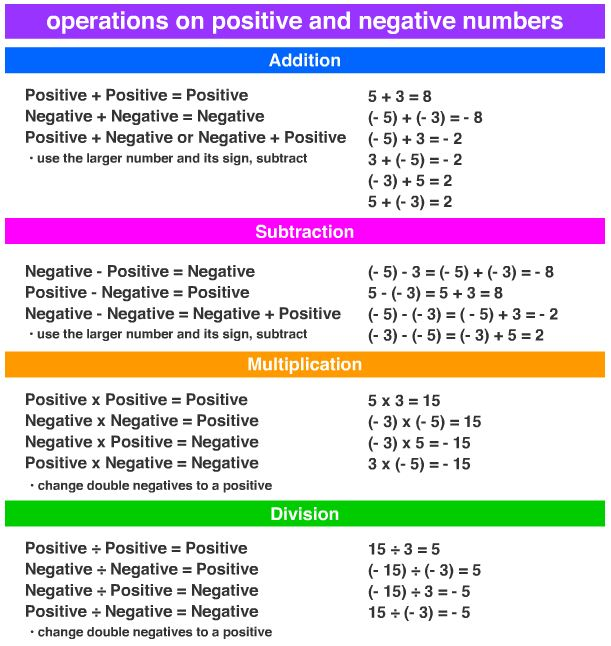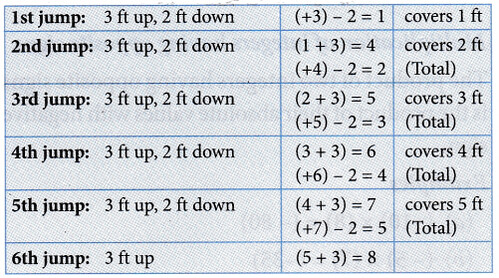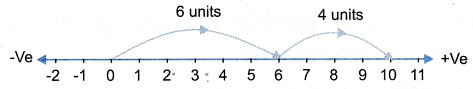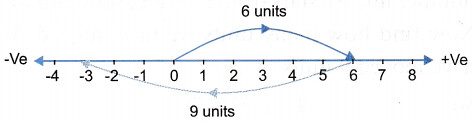# Fundamental Operations on Integers

## Fundamental Operations on Integers

We have four fundamental operations on integers. They are addition, subtraction, multiplication, and division.A monkey is sitting at the bottom in an empty water tank 8 ft high. The monkey wants to jump to the top of the water tank. He jumps 3 ft up and then slides 2 ft down. In how many jumps will the monkey reach the top of the empty water tank?

Monkey’s jump:The monkey will reach the top of the empty water tank in the 6th jump.
Two integers can be added in the same way as two whole numbers are added but while adding negative integers, we have to move to the left on the number line.

Addition of integers having the same sign
1. The sum of two positive integers is the sum of their absolute values with a positive sign.

Example 1: Add (+ 6) + (+4).
Solution: On a number line, first draw an arrow from 0 to 6 and then go 4 steps ahead. The tip of the last arrow reaches +10. So, (+ 6) + (+ 4) = +102. The sum of two negative integers is the sum of their absolute values with negative sign(-).

Example 2: Add (-3) + (-4).
Solution: On a number line, first we draw an arrow on the left side of zero from 0 to -3 and then further move to the left 4 steps. The tip of the last arrow is at -7. So, (-3) + (-4) = (-7)Addition of integers having opposite signs
The sum of two integers having opposite signs is the difference of their absolute values with the sign of integer of greater absolute value.

Solution: On a number line, first we draw an arrow from 0 to 6 on the right and then go 9 steps to the left. The tip of the last arrow is at -3. So, (+6) + (-9) = (-3)### 2. Subtraction of integers

In subtraction, we change the sign of the integer which is to be subtracted and then add to the first integer. In other words, if a and b are two integers, then a – b = a + (-b)

Example 4: Subtract 5 from 12.
Solution: (12) – (5) = (12) + (-5) = 7Example 5: Subtract -7 from -15.
Solution: (-15) – (-7) = (-15) + (7)= -8Example 6: Subtract 6 from -10.
Solution: (-10) -(6) = (-10) + (- 6)Example 7: Subtract (-5) from 4.
Solution: 4 – (-5) = 4 + (5) = 9To subtract (-5) from 4, we have to find a number which when added to (-5) gives us 4. So, on the number line we start from (-5) and move up to 4. Now find how many units we have moved. We have moved 9 units.
So, 4-(-5) =9

Note:

(a) The sum of two integers with like signs is the sum of their absolute values with the same sign.
(b) The sum of two integers with unlike signs is the difference of their absolute values with _the sign of the greater absolute value.
2. Subtraction of integers
The sign of the integer is changed which is to be subtracted and then added to the first integer.

### 3. Multiplication of integers

Multiplication of integers having the same sign
When two integers have the same sign, their product is the product of their absolute values with positive sign.
Examples
(a) (+6) × (+7) = + 42 or 42
(b) (+5) × (+10) = + 50 or 50
(c) (-3) × (-5) = + 15 or 15
(d) (-20) × (-6) = 120
(e) (12) × (5) = 60

Multiplication of integers having opposite signs
The product of two integers having opposite signs is the product of their absolute values with negative sign.
Examples
(a) (-10) × (8) = (- 80)
(b) (- 5) × (7) = (-35)
(c) (12) × (-3) = (-36)
(d) (-6) × (3) = (-18)
(e) 5 × (-4) = (-20)

Note:
plus × minus = minus
minus × plus = minus
minus × minus = plus
plus × plus = plus

### 4. Division of integers

Division of integers having the same sign
Division of two integers having the same sign is the division of their absolute value with a positive sign. If both integers have the same sign, then the quotient will be positive.
Examples:
(a) (+9) ÷ (+3) = (3)
(b) (-9) ÷ (-3) = (3)
(c) (-24) ÷ (-12) = (2)

Division of integers having opposite signs
If both integers have different signs, the quotient will be negative.
Examples: (a) 12 ÷ (-3) = (-4)
(b) (-10) ÷ (5) = (-2)
(c) (-18) ÷ (3) = (-6)

Example 8: Evaluate (-13) – (-7 – 6).
Solution: (-13) – (-7 – 6)
= (-13) -(-13)
= (-13) + (13) (Opposite to each other) = 0

Example 9: Subtract (-5128) from 0.
Solution: 0 – (-5128) = 0 + 5128 = 5128

Example 10: Divide (4000) + (- 100).
Solution: $$\frac{4000}{-100}$$ = -40

Example 11: Multiply (-18) and (-8).
Solution: (-18) × (-8) = 18 × 8 = 144

Note:

1. Multiplication of integers
(a) When two integers have the same sign, their product is the product of their absolute values with a positive sign.
(b) The product of two integers having opposite signs is the product of their absolute values with a negative sign.
2. Division of integers
(a) If integers have the same sign, the quotient is always positive.
(b) If integers have opposite signs, the quotient will be negative.

Note:

• The integers are …, -3,-2,-1, 0,1, 2, 3,…
• 1, 2, 3, 4,… are called positive integers and -1,-2,-3,… are called negative integers. 0 is neither positive nor negative.
• Integer 0 is less than every positive integer but greater than every negative integer.
• The absolute value of an integer is the numerical value of the integer regardless of its sign.
• The absolute value of an integer is either positive or zero. It cannot be negative.
• The sum of two integers having the same sign is the sum of their absolute values with a positive sign.
• The sum of two integers having opposite signs is the difference of their absolute values with the sign of the greater absolute value.
• To subtract an integer b from a we change the sign of b and add, i.e., a + (-b)
• The product of two integers having the same sign is positive.
• The product of two integers having different signs is negative.
• Two integers, which when added give 0, are called additive inverse of each other.
• Additive inverse of zero is 0.